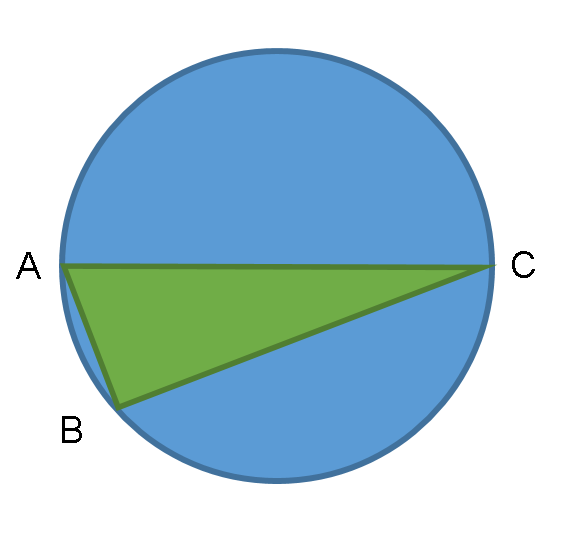Triangle in circle

Geometry Level 4In the diagram above, AC represents the diameter, B lies on the circumference of the circle, and you are given that (Area of Circle) / (Area of Triangle) = 2π. What is the difference, in degrees, of the two smaller angles of the triangle?

×